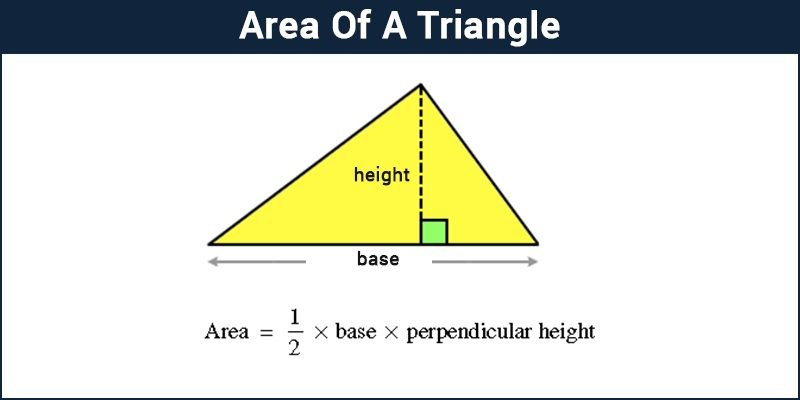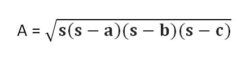# Area of a Triangle

The area of a triangle is defined as the total space that is enclosed by that particular triangle. The basic formula to find the area of a triangle is A = 1/2 × b × h, where b is the base and h is the height of the given triangle.

Example of Area of Triangle:

• To find the area of the triangle with base b as 3 cm and height h as 4 cm, we will use the formula for
• Area of a Triangle – A = 1/2 × b × h = 1/2 × 4cm × 3cm = 2cm × 3cm = 6cm2

In general, the term “area” is defined as the region occupied inside the boundary of a flat object or figure. The measurement is done in square units with the standard unit being square meters (m2). For the computation of area, there are pre-defined formulas for squares, rectangles, circle, triangles, etc.

In this article, we would discuss the area of a triangle. For this, we would use the area of a triangle formulas on different types of triangles and see if we are able to obtain their area.## Area and Perimeter of a Triangle Formula

The area of the triangle  is given by the formula mentioned below:

• Area of a Triangle = A = ½ (b × h) square units
• The perimeter of a triangle = P = a + b + c units,

where a, b, and c are the three sides of a triangle

### How to Calculate Area of Triangle

Now, let’s see how to calculate the area of a triangle using the given formulas. The area formulas for all the different types of triangles like an area of an equilateral triangle, right-angled triangle, an isosceles triangle are given below.

• Area of a Right Triangle = A = ½ × Base × Height
• Area of an Equilateral Triangle = A = (√3)/4 × a2
• Area of an Isosceles Triangle = A = ½ (b × h),

where,

b is base and h is the height of the triangle,

a is the side of the triangle.

## Area of a Triangle with Three Sides

The area of a triangle with three sides can be found using Heron’s formula if all the three sides of the triangle are given. Heron’s formula needs the measurement of all three sides of the triangle as it is used to find the semiperimeter first and then using the same in the main formula to find the area of the triangle., where s is semi-perimeter = (a+b+c) / 2

We have seen that the area of special triangles could be obtained using the triangle formula. However, for a triangle with the sides being given usually, calculation of height would not be simple. For the same reason, we rely on Heron’s Formula to calculate the area of the triangles with unequal lengths.

### Area of  Triangle problems

Example 1: Find the area of the acute triangle with a base of 13 inches and a height of 5 inches.

Solution:

A = (½)× b × h sq.units

⇒ A = (½) × (13 in) × (5 in)

⇒ A = (½) × (65 in2)

⇒ A = 32.5 in2

Example 2: Find the area of the right-angled triangle with a base of 7cm and a height of 8cm.

Solution:

A = (½) × b × h sq.units

⇒ A = (½) × (7 cm) × (8 cm)

⇒ A = (½) × (56 cm)

⇒ A = 28 cm2

Example 3: Find the area of obtuse-angled triangle with a base of 4cm and a height 7cm.

Solution:

A = (½) × b × h sq.units

⇒ A = (½) × (4 cm) × (7 cm)

⇒ A = (½) × (28 cm)

⇒ A = 14 cm2